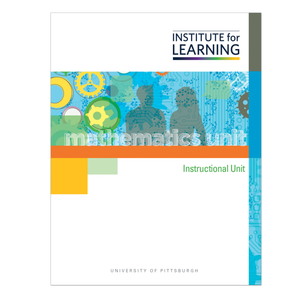# Grade 5: Division with Fractions (CCSSM Version)

Regular price \$149.00 \$0.00 Unit price per

Concepts

• Divide a Whole Number, a, by a Whole Number, b
• Divide a Whole Number by a Unit Fraction
• Divide a Unit Fraction by a Whole Number

Unit includes

• 12 high-level tasks with associated QuickStarts, Practices, Applications, and Quick Writes.
• 3 checkpoints and 6 support activities.
• 1 final performance-based assessment with scoring guide.

Students

• Explore and solve division situations involving fractions, including cases in which a fraction is the quotient, cases in which a fraction is the divisor, and cases in which a fraction is the dividend.
• Make sense of and create representations that show how many of the unit fractions are in each of the whole numbers.
• Make sense of and explain what happens when a fraction is partitioned by a whole number.
• Identify and explain the difference between dividing a whole number by a unit fraction and dividing a unit fraction by a whole number.